# Tomatoes

There were 2414 crates of tomatoes in the barn, but 735 crates of tomatoes were rotten and had to be thrown out. Joe sold 813 crates and canned 756 crates of tomatoes. How many crates of tomatoes does Joe have left?

n =  110

### Step-by-step explanation:

$n=2414-735-813-756=110$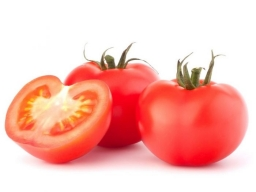Did you find an error or inaccuracy? Feel free to write us. Thank you!## Related math problems and questions:

• ConcertOn a Concert were sold 150 tickets for CZK 360, 235 tickets for 240 CZK and 412 for 180 CZK. How much was the total revenues for tickets?
• RemaindersIt is given a set of numbers { 170; 244; 299; 333; 351; 391; 423; 644 }. Divide this numbers by number 66 and determine set of remainders. As result write sum of this remainders.
• There 14There are 250 people in a museum. 2/5 of the 250 people are girls 3/10 of the 250 people are boys The rest of the 250 people are adults Work out the number of adults in the museum.
• I think numberI think number.When I add 841 to it and subtract 157, I get a number that is 22 greater than 996. What number I thinking?
• Crates 2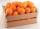One crate will hold 50 oranges. If Bob needs to ship 932 oranges, how many crates will he need?
• The percentages in practiceIf every tenth apple on the tree is rotten it can be expressed by percentages: 10% of the apples on the tree is rotten. Tell percent using the following information: a. in June rained 6 days b, increase worker pay 500 euros to 50 euros c, grabbed 21 from
• Four prismsQuestion No. 1: The prism has the dimensions a = 2.5 cm, b = 100 mm, c = 12 cm. What is its volume? a) 3000 cm2 b) 300 cm2 c) 3000 cm3 d) 300 cm3 Question No.2: The prism base is a rhombus with a side length of 30 cm and a height of 27 cm. The height of t
• Voting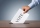Four hundred employees cast their votes in a board member election that has only two candidates. One hundred twenty people vote for candidate A, while half of the remaining voters abstain. How many votes does candidate B receive?
• Candies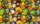There are 150 candies in the bowl. Ivan took 7 thirtieths, Charles 6 fifteenths, and Thomas 3 tenths. How many sweets are left in the bowl?
• Divisible by 5How many three-digit odd numbers divisible by 5, which are in place ten's number 3?
• SchoolThere are 150 pupils in grade 5 . 2/3 of them are female. By what fractions are the males?
• RatiosReduce the numbers: 50 in a 1:2 ratio 111 at a ratio of 2:3 70 at 10:50 560 at a ratio of 3:8
• How manyHow many integers are greater than 547/3 and less than 931/4?
• School year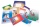At the beginning of the school year, 396 notebooks and 252 textbooks are ready to be distributed in the classroom. All pupils receive the same number of notebooks and the same amount of textbooks. How many pupils are there in the class if you know that th
• Infants water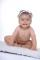The tank, which has 10 hectoliters of water for infants, went 580 liters in the morning and 340 liters in the afternoon. How many liters left in the tank?
• Students at cinema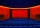All pupils from the school went to the cinema for a film performance. The cinema has 400 seats, 2 rows of 20 seats remain unoccupied. Teachers occupied 10 places. How many students were there?
• Cash back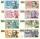After a trip 24 students have left more than 650 but less than 690 CZK (Kc). How much each student receives return when the amount is divisible by the number of pupils?